# PyDPF-Post feature examples#

These examples show how you use PyDPF-Post features.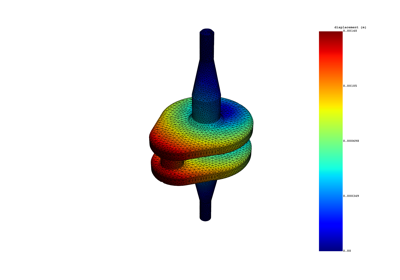Create and manipulate a DPF Dataframe

Create and manipulate a DPF Dataframe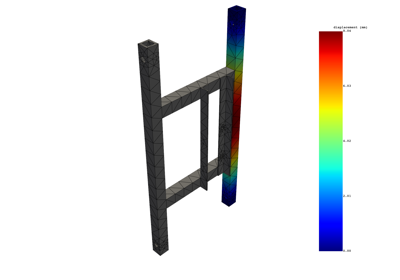Extract results on named selections - Modal Simulation

Extract results on named selections - Modal Simulation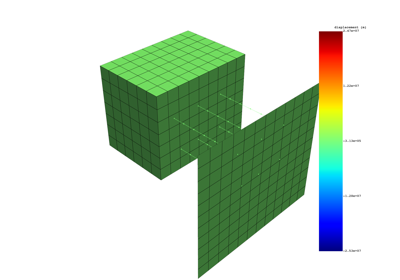Extract components of results - Modal Simulation

Extract components of results - Modal Simulation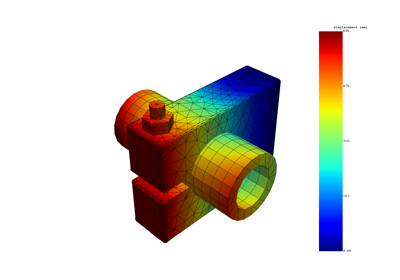Explore the data of a result with the DataFrame - Harmonic Simulation

Explore the data of a result with the DataFrame - Harmonic Simulation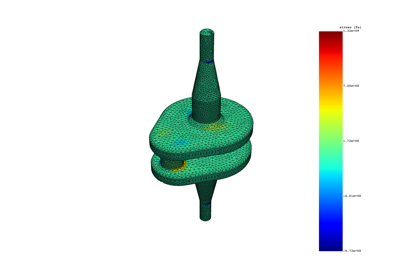Extract stress/strain invariants - Static Simulation

Extract stress/strain invariants - Static SimulationExport data contained in a DataFrame to a new format

Export data contained in a DataFrame to a new format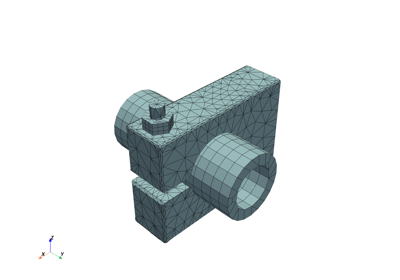Explore the mesh

Explore the meshCompute minimum and maximum of a DataFrame

Compute minimum and maximum of a DataFrame